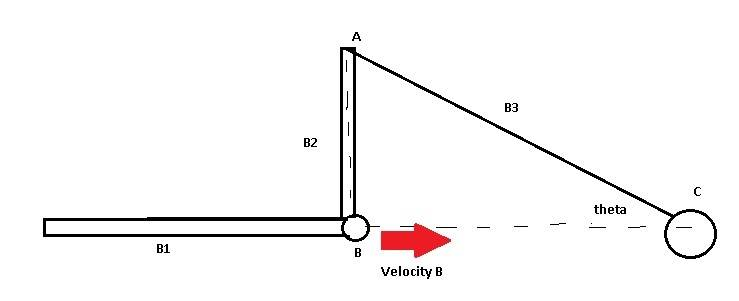# Quick question about centers of instantaneous velocities

## Homework StatementIf at some time t, the position of B (the intersection between bodies B1 and B2) is located directly below the connection between bodies B2 and B3. B is moving to the right at a speed of Vb. What happens to the instantaneous center of B2, and what can this tell you about the angular velocity of B3? (Note: body B3 is pinned at the location C and B is restricted to move in the horizontal direction). Explain.

## Homework Equations

Velocity of the instant center is 0.
v = rw, where v = velocity, r = distance to the point, and w = angular velocity

## The Attempt at a Solution

Since the perpendicular path of the velocity of B passes through point A, and the velocity of A is restricted to be moving tangent to the circular path around C, the only thing that makes sense to me is if B3 has an angular velocity to be 0 and the velocity at A is zero. The reason why is because at any moment prior to the instant that B2 is vertical, the angular velocity of B3 has to be in one direction since theta is growing, but as the body becomes more and more vertical, the magnitude of this velocity decreases until it has to reach a zero point since any point following this instant will have the angular velocity begin to change in the opposite direction (theta will be getting smaller).

I am a little concerned that my reasoning here may be off though, or that I'm missing something important.

•